# Multiply Matrix by Vector in R

A matrix is a 2-dimensional structure whereas a vector is a one-dimensional structure. In this article, we are going to multiply the given matrix by the given vector using R Programming Language. Multiplication between the two occurs when vector elements are multiplied with matrix elements column-wise.

Approach:

• Create a matrix
• Create a vector
• Multiply them
• Display result.

Method 1: Naive method

Once the structures are ready we directly multiply them using the multiplication operator(*).

Example:

## R

 `# create a vector for matrix elements ` `vector1=``c``(1,2,3,4,5,6,7,8,9,10,11,12) ` ` `  `# Create A matrix with 2 rows and 6 columns ` `matrix1 <- ``matrix``(vector1, nrow=2,ncol=6)  ` ` `  `# multiplication vector ` `mul_vec=``c``(1,2,3,4) ` ` `  `# print multiplication result ` `print``(matrix1*mul_vec)`

Output: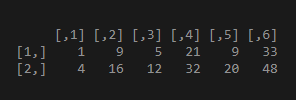Example 2:

## R

 `# create a vector for matrix elements ` `vector1=``c``(1,2,3,4,5,6,7,8,9,10,11,12,13,14,15,16) ` ` `  `# Create A matrix with 4 rows and 4 columns ` `matrix1 <- ``matrix``(vector1, nrow=4,ncol=4)  ` `print``(matrix1) ` ` `  `mul_vec=``c``(1,2,3,4) ` ` `  `print``(``"Result"``) ` `print``(matrix1*mul_vec) `

Output: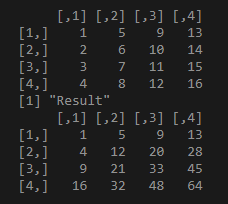Example 3:

This code has both matrix and vector has equal size

## R

 `# create a vector for matrix elements ` `vector1=``c``(1,2,3,4) ` ` `  `# Create A matrix with 2 rows and 2 columns ` `matrix1 <- ``matrix``(vector1,nrow=2,ncol=2)  ` `print``(matrix1) ` ` `  `mul_vec=``c``(1,2,3,4) ` ` `  `print``(``"Result"``) ` `print``(matrix1*mul_vec) `

Output: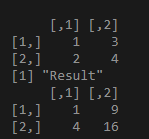Method 2: Using sweep()

we can use sweep() method to multiply vectors to a matrix. sweep() function is used to apply the operation “+ or – or ‘*’ or ‘/’ ” to the row or column in the given matrix.

Syntax:

sweep(data, MARGIN,  FUN)

Parameter:

• data=input matrix
• MARGIN: MARGIN = 2 means row; MARGIN = 1 means column.
• FUN: The operation that has to be done (e.g. + or – or * or /)

Here, we are performing “*” operation

Example:

## R

 `# create matrix with 15 elements ` `matrix1 <- ``matrix``(``c``(1,2,3,4,5,6,7,8,9,10,11,12,13,14,15), ` `                  ``nrow=3,byrow=``TRUE``) ` `print``(matrix1) ` `print``(``"---------------"``) ` ` `  `# create a vector ` `vector1 <- ``c``(1,2,3,4,5) ` ` `  `# apply sweep operation that multiplies row  ` `# wise(margin=2) ` `print``(``sweep``(matrix1, MARGIN=2,vector1, `*`)) ` `print``(``"---------------"``) ` ` `  `# create elements with vector 2 ` `vector2 <- ``c``(1,2,3) ` ` `  `# apply sweep operation that multiplies column ` `# wise with vector 2(margin=1) ` `print``(``sweep``(matrix1, MARGIN=1,vector2, `*`))`

Output: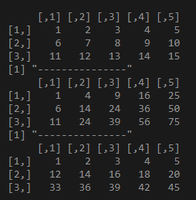Example 2:

## R

 `# create matrix with 8 elements ` `matrix1 <- ``matrix``(``c``(1,2,3,4,5,6,7,8), ` `                  ``nrow=2,byrow=``TRUE``) ` `print``(matrix1) ` `print``(``"---------------"``) ` ` `  `# create a vector ` `vector1 <- ``c``(1,2,3,4) ` ` `  `# apply sweep operation that multiplies  ` `# row wise(margin=2) ` `print``(``sweep``(matrix1, MARGIN=2,vector1, `*`)) ` `print``(``"---------------"``) ` ` `  `# create elements with vector 2 ` `vector2 <- ``c``(1,2) ` ` `  `# apply sweep operation that multiplies column  ` `# wise with vector 2(margin=1) ` `print``(``sweep``(matrix1, MARGIN=1,vector2, `*`))`

Output: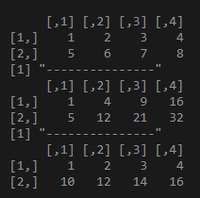Whether you're preparing for your first job interview or aiming to upskill in this ever-evolving tech landscape, GeeksforGeeks Courses are your key to success. We provide top-quality content at affordable prices, all geared towards accelerating your growth in a time-bound manner. Join the millions we've already empowered, and we're here to do the same for you. Don't miss out - check it out now!

Previous
Next